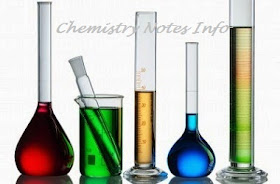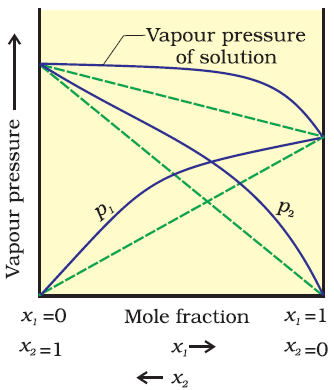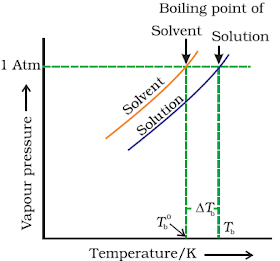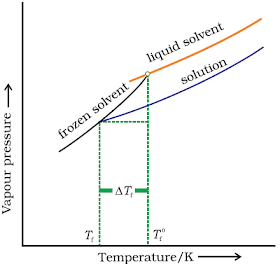## SOLUTION

### Solution: -A solution is homogeneous mixture of two or more chemically non-reacting substances, whose composition can be varied within certain limits.

### Expressing concentration of solutions

#### 1.      Percentage:-

=>  mass percentage (%) of a component  (W/W)

= Mass of the component in solution*100 / Total mass of the solution

=>  volume percentage (%) of the component (V/V)
= Volume of the component * 100 / Total volume of the solution
=>  Mass/Volume percentage (%) of the component (W/V)
=Mass of the component in solution * 100 / Total volume of the solution

#### 2.      Strength:-

Strength of the solution ( gl-1 or gdm-3)
= Mass of the solute in grams / Volume of the solution in litre

### 3.      Molarity (M) :-

No. of moles of the solute  / Volume of the solution in litre
M = strength in gram per litre / Molar mass of the solute

### 4.      Mole fraction :-

It is obtained by dividing the no. of moles of the solute or solvent by the total no. of moles of the solution.
Mole fraction,    X1 = n1/n1+n2
X2 = n2/n1+n2
n1 = no. of moles of the solvent
n2 = no. of moles of the solute
X1+X2 = 1

#### 5.      Mass fraction: -

Mass of the given component per unit mass of the solution denoted by X.
XA = WA / WA+WB
XB = WB / WA+WB
WA = Mass of the solvent
WB = Mass of the solute
XA+XB = 1

### Solution of solids in liquids

Solubility of a solid in a liquid :- It is defined as the maximum amount of the solid (solute) in grams which can dissolve in 100 grams of liquid (solvent) to form the saturated solution at the particular temperature.

#### Factors affecting the solubility of a solid in a liquid

i.            Nature of the solute & the solvent :- “Like dissolves Like”
=> The polar (ionic) compounds like NaCl dissolve in polar solvent like water.
=>  The non polar (covalent or organic) compounds dissolve in non polar compounds like Anthracene dissolve in Benzene.
ii.            Effect of temperature :-
=>  The solubility increases with increase of temperature when the process of dissolution is endothermic.
Solute + Solvent + Heat -> Solution
Ex:- NaNo3, KNO3, NaCl, KCl
=>  The solubility Decreases with increase in temperature when the process of dissolution is exothermic.
Solute + Solvent -> Solution + Heat
Ex:- Sodium carbonate mono hydrate (Na2CO3.H2O).
=>  Those whose solubility does not increases or decreases continuously
Ex:- CaCl2.6H2O -> CaCl2.4H2O -> CaCl2.2H2O

### Solution of Gases in liquids

1)      Solubility of a gas in a liquid :- The solubility of a gas in a particular liquid is the volume of the gas in CC’s (centimetre cubes CM3) that can dissolve in unit volume of the liquid to form the saturated solution at the temperature of the experimented under a pressure of one atmosphere.

#### Factors affecting the solubility of a liquid in a gas

i)        Nature of the gas & the solvent:- Gases like Hydrogen, Oxygen, Nitrogen etc. dissolve in water only to a small extent but gases like CO2, HCl, NH3 are highly soluble.
The greater solubility of later gases they react with solvent. the greater solubility of the gas in a solvent due to their chemical similarity.

ii)      Nature of temperature:- The solubility of gases decreases with increase  in temperature.
iii)    Effect of pressure (Henry’s Law):- By increasing the pressure solubility also increases.

### Henry’s Law:-

The mass of a gas dissolved in the given volume of liquid at constant temperature is directly proportional to the pressure of the gas present in equilibrium with the liquid.
m p
m=KHP
Also,
The partial pressure of gas in vapour phase (P) is proportional to the mole fraction of gas (x) in the solution.
P=KHX

#### Limitations of Henry’s law

Henry’s law is applicable only if the the following conditions are satisfied:-
a)      Pressure should be low and temperature should be high i.e. gas behaves as an ideal gas.
b)      The gas should not go under compound formation with solvent or association or dissociation in the solvent.

#### Vapour Pressure of liquid solution:-

Vapour pressure of liquid/solution is the pressure exerted by the vapour in equilibrium with the liquid/solution at a particular temperature.

### Raoults Law:-

It states that for a solution of volatile liquids, the partial vapour pressure of each component in the solution is directly proportional to its mole fraction.

For component A
PA XA
PA = P0AXA
Where, P0A = Vapour pressure of pure component A at the same temperature

For component B,
PB = P0BXB

#### By Daltons law of partial pressure:-

Ptotal = PA+ PB
Ptotal = P0AXA + P0BXB
= (1-XB) P0A+ P0BXB
= P0A - P0AXB + P0BXB
= P0A+ (P0B- P0A)XB
If YA  & YB represents the mole fraction of component A & B respectively.

In vapour phase,
YA = PA/ PA+ PB = PA/ Ptotal         ……………………..(i)
YB = PB/ PA+ PB = PB/ Ptotal         ……………………..(ii)
From eq. (i) & (ii)………
PA = YA * Ptotal
PB = YB * Ptotal
In general,
Pi = Yi * Ptotal

### Raoults Law for non volatile liquid:-

Vapour pressure of each component is directly proportional to its mole fraction.
P1  µ X1
P1 = P01X1
P1/P01 = X1

### Ideal solutions:-

An ideal is that solution which obeys raoults law in all conditions of temperature an concentration.
i.e. ∆ Vmixing = 0
∆ Hmixing = 0
Also, forces of attraction between components are same as in pure state.
A-A = A-B = B-B

### Non ideal solutions:-

The solutions which not obeys the Raoults Law is known as non ideal solutions.
∆ Vmixing ≠ 0, ∆ Hmixing ≠ 0
v  The force of interaction between solvent/solute is different than in pure state.

### Positive deviation:-Positive Deviation

In this A-B interaction are weaker than A-A, B-B
i.e. interaction between solute-solvent is weaker than solute-solute or solvent-solvent. This means in such solutions the molecules of A or B (A-B) will find it easier to escape than in pure state.

### Negative deviation:-Negative Deviation

Intra molecular attraction force between A-A and B-B are weaker than those between A-B. this decreases the escaping tendency of molecules for each component (A-B) and consequently the vapour pressure decreases resulting in –Ve deviation from raoults law.

### Colligative Properties: -

Those properties of ideal solutions which depends only on the no. of particles of the solute dissolve in the definite amount of solvent and do not depend upon nature of solute.
The important colligative properties are:-

#### Relative lowering of vapour pressure: -

from Raoults law for solutions of solids in liquids.
Where, P0 = vapour pressure of pure solvent
PS = vapour pressure of solution
n2 = no. of moles of solute
n1 = no. of moles of solvent
For dilute solutions n2<<n1
Hence, neglecting n2 in the denominator we have,
Here, w1 & w2 are the masses and m1 & m2 are masses of solvent & solute respectively.

### Osmotic Pressure:-

The process of the flow of the solvent molecules from solvent to solution through semi permeable membrane (SPM) is called osmosis the flow of the solvent molecules can be stopped if some extra pressure is applied on the solution. This pressure that just stops the flow of solvent molecules is called osmotic pressure of the solution.
=>> Osmotic pressure of the solution is directly proportional to the molar concentration or molarity (C) of the solution and its temperature (T).
Mathematically,
Or,                      π = R*C*T
Where, R is solution constant equal to the gas constant
π = CRT
Hence,         π = (n2/v) RT
this equation is called von’t hoff equation for dilute solutions.

### Elevation in boiling point:-

It is found that the boiling point of the solution is always higher than that of pure solvent. The increase is called elevation in boiling point.Elevation in boiling point
From figure,   we known       ∆Tb = Tb - T0b
Where, T0b  & Tb is the temperature at which the vapour pressure of the solvent and solution becomes equal to the atmospheric pressure and ∆Tb is called elevation in boiling point.
Also, ∆Tb is directly proportional to m
∆Tb = kbm
Where, kb is called molal elevation constant or ebullioscopy constant and ‘m’ is the molality of the solution.

#### =>  Calculation of molecular mass of the solute:-

As molality is the no. of moles of the solute dissolve per 100 gm of the solvent. If w2 grams of the solute of molecular mass m2 are dissolve in w1 gram of the solvent
m = (W2/M2)(1000/W1)

### Depression in freezing point:-

It is observed that the freezing point of the solution is always lower than that of pure solvent. And the decrease is called the depression in freezing point.Depression in freezing point

From figure,     ∆Tf = T0f –Tf
Where,  T0f = freezing point of pure solvent
Tf = freezing point of solution
∆Tf = Depression in freezing point

Also,
∆Tf  is directly proportional to m
∆Tf  = kf m
Where, kf is molal depression constant or cryoscopic constant and m is molality of the solution.

#### =>  Calculation of molecular mass of solute:-

∆Tf = Kf(w2/m2)(1000/W1)

### Vant hoff factor:-

It is defined as the ratio of the experimented value to the calculated property of the colligative property known as vant hoff factor.